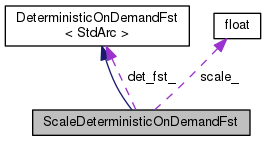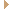ScaleDeterministicOnDemandFst Class Reference

Class ScaleDeterministicOnDemandFst takes another DeterministicOnDemandFst and scales the weights (like applying a language-model scale). More...

`#include <deterministic-fst.h>`

Inheritance diagram for ScaleDeterministicOnDemandFst:[legend]
Collaboration diagram for ScaleDeterministicOnDemandFst:[legend]

## Public Types

typedef StdArc::Weight Weight

typedef StdArc::StateId StateId

typedef StdArc::Label LabelPublic Types inherited from DeterministicOnDemandFst< StdArc >
typedef StdArc ::StateId StateId

typedef StdArc ::Weight Weight

typedef StdArc ::Label Label

## Public Member Functions

ScaleDeterministicOnDemandFst (float scale, DeterministicOnDemandFst< StdArc > *det_fst)

StateId Start ()

Weight Final (StateId s)

bool GetArc (StateId s, Label ilabel, StdArc *oarc)Public Member Functions inherited from DeterministicOnDemandFst< StdArc >
virtual Weight Final (StateId s)=0

virtual bool GetArc (StateId s, Label ilabel, StdArc *oarc)=0
Note: ilabel must not be epsilon. More...

virtual ~DeterministicOnDemandFst ()

## Private Attributes

float scale_

DeterministicOnDemandFst< StdArc > & det_fst_

## Detailed Description

Class ScaleDeterministicOnDemandFst takes another DeterministicOnDemandFst and scales the weights (like applying a language-model scale).

For instance, to subtract existing LM scores from a lattice you could use this with a negative weight; and to interpolate LMs you can also use this with weights less than one.

It's specialized for StdArc because there is no generic way to scale weights.

Definition at line 129 of file deterministic-fst.h.

## ◆ Label

 typedef StdArc::Label Label

Definition at line 133 of file deterministic-fst.h.

## ◆ StateId

 typedef StdArc::StateId StateId

Definition at line 132 of file deterministic-fst.h.

## ◆ Weight

 typedef StdArc::Weight Weight

Definition at line 131 of file deterministic-fst.h.

## ◆ ScaleDeterministicOnDemandFst()

 ScaleDeterministicOnDemandFst ( float scale, DeterministicOnDemandFst< StdArc > * det_fst )
inline

Definition at line 136 of file deterministic-fst.h.

137  :
138  scale_(scale), det_fst_(*det_fst) { }
DeterministicOnDemandFst< StdArc > & det_fst_

## ◆ Final()

 Weight Final ( StateId s )
inline

Definition at line 142 of file deterministic-fst.h.

142  {
143  // Note: Weight is indirectly a typedef to TropicalWeight.
144  Weight final = det_fst_.Final(s);
145  if (final == Weight::Zero()) return Weight::Zero();
146  else return TropicalWeight(final.Value() * scale_);
147  }
DeterministicOnDemandFst< StdArc > & det_fst_
virtual Weight Final(StateId s)=0

## ◆ GetArc()

 bool GetArc ( StateId s, Label ilabel, StdArc * oarc )
inline

Definition at line 149 of file deterministic-fst.h.

149  {
150  if (det_fst_.GetArc(s, ilabel, oarc)) {
151  oarc->weight = TropicalWeight(oarc->weight.Value() * scale_);
152  return true;
153  } else {
154  return false;
155  }
156  }
DeterministicOnDemandFst< StdArc > & det_fst_
virtual bool GetArc(StateId s, Label ilabel, Arc *oarc)=0
Note: ilabel must not be epsilon.

## ◆ Start()

 StateId Start ( )
inlinevirtual

Implements DeterministicOnDemandFst< StdArc >.

Definition at line 140 of file deterministic-fst.h.

140 { return det_fst_.Start(); }
DeterministicOnDemandFst< StdArc > & det_fst_
virtual StateId Start()=0

## ◆ det_fst_

 DeterministicOnDemandFst& det_fst_
private

Definition at line 160 of file deterministic-fst.h.

## ◆ scale_

 float scale_
private

Definition at line 159 of file deterministic-fst.h.

The documentation for this class was generated from the following file: